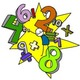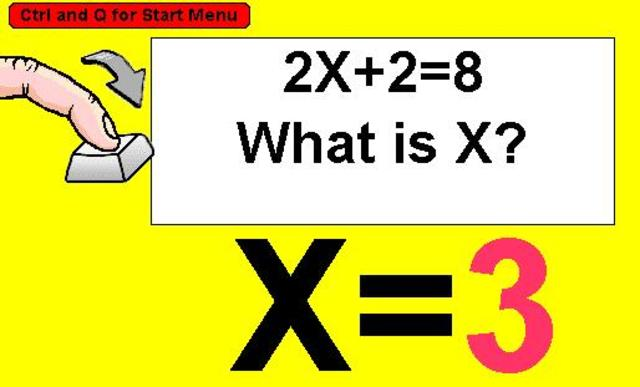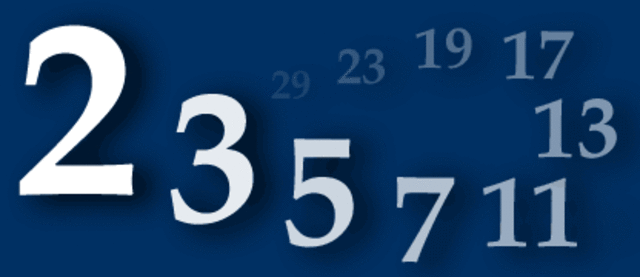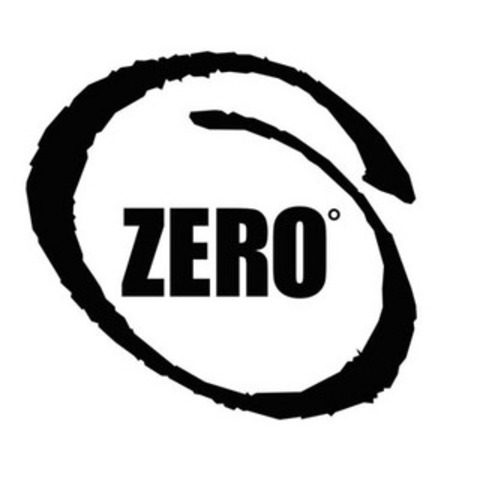• 200

# Numbering in Egypt using 1's, 10's, 100's etcAbout 7000 years ago, the Egyptians started to use a number system linked to units, tens, hundreds etc.
• 200

# old things thousands of years before Christ7000 years ago Babylonians use multiplication tables
They also use Pythagoras's Theorem 4000 years ago , and can solve quadratic equations. But they still don't even have any 0's (Zero) for another 2000 years.
• Jan 1, 1225

# Letters used in algebraLetters seem to have been first used instead of numbers in algebra, to represent numbers which we don't know. Sometimes these letters represent fixed numbers (constants) and sometimes they can be chosen (variables)
• Jan 1, 1248

# Negative NumbersLi Yeh writes a book which contains negative numbers, denoted by putting a diagonal stroke through the last digit. We now use the -sign e.g. -18
• Jan 1, 1492

# Decimal PointFrancesco Pellos wrote a commerical arithmetic book, Compendio de lo abaco, in which he made use of a dot to denote the divison of an integer by a power of ten. This led to the development of what we now refer to as a decimal point.
• Jan 1, 1557

# The equals signRobert Recorde parallel lines (=) to represent the equals sign for the first time
• # First calculating machineA mathematician called Schickard makes a wooden calculating machine that can add and subtract, and help with multiplication and division.
• # Hand held calculatorA Texas Instuments developed a calculator small enough to be held in your hand. The previous calculat whighed as much an average year 8 pupil!
• # prime numbers used for codesAdleman, Rivest, and Shamir introduce public-key codes, a system for passing secret messages using large prime numbers. This is the basis of security for bank cards and internet shopping.
• # Zero (O) is recognised and usedZero is used. Before this there wasn't a symbol for Zero. There were already marks used to represent a space in one of the collumns (like the 0 in 2012), but there had not been a symbol for none (like zero sheep)